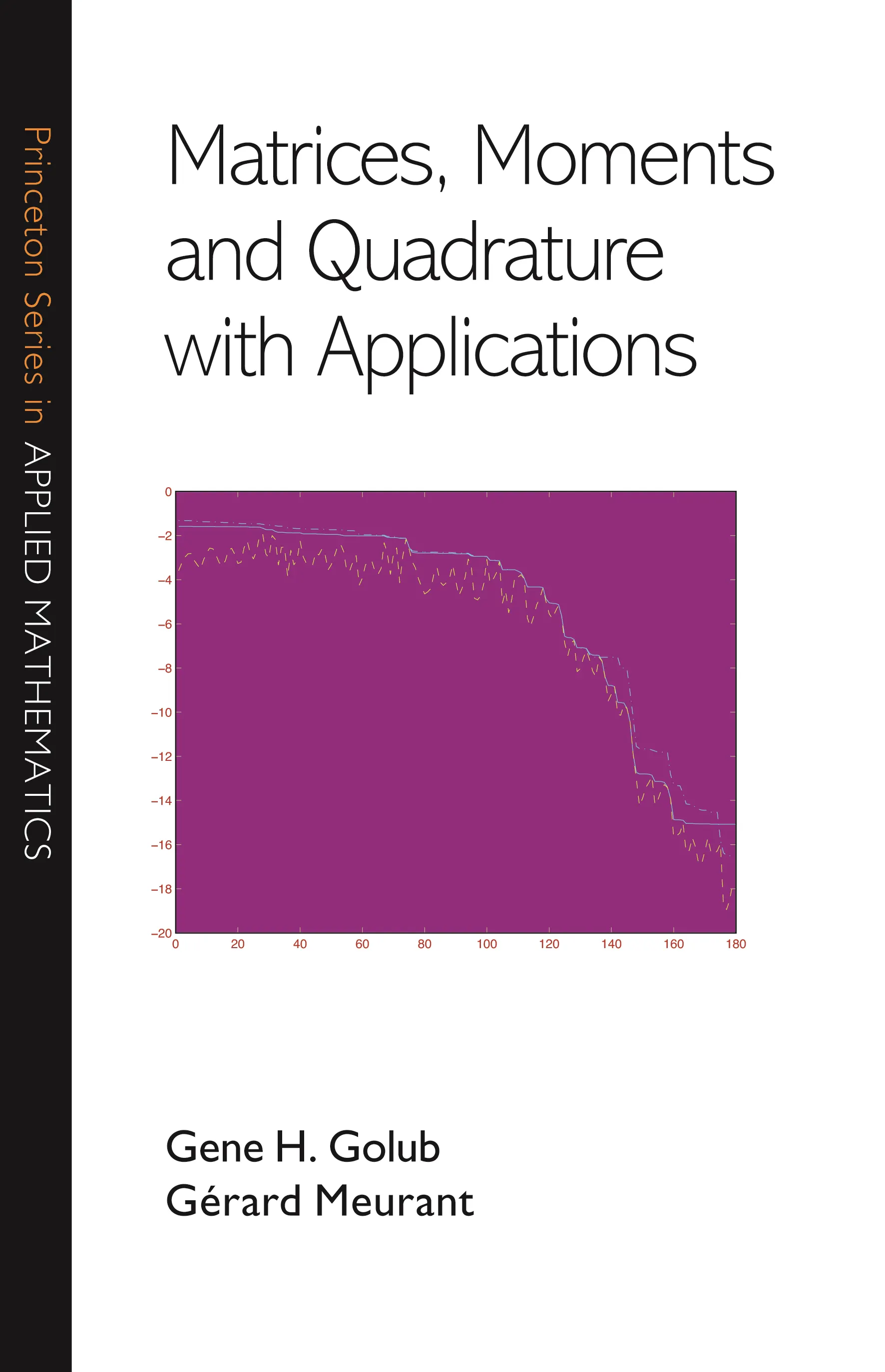#### Matrices, Moments and Quadrature with Applications

Gene H. Golub

##### ebooks

Many of our ebooks are available for purchase from these online vendors:

Many of our ebooks are available through library electronic resources including these platforms:

# Matrices, Moments and Quadrature with Applications## Hardcover

Price:
\$90.00 / £70.00
ISBN:
Published:
Dec 27, 2009
2010
Pages:
376
Size:
6 x 9.25 in.
Illus:
88 line illus. 135 tables.

This computationally oriented book describes and explains the mathematical relationships among matrices, moments, orthogonal polynomials, quadrature rules, and the Lanczos and conjugate gradient algorithms. The book bridges different mathematical areas to obtain algorithms to estimate bilinear forms involving two vectors and a function of the matrix. The first part of the book provides the necessary mathematical background and explains the theory. The second part describes the applications and gives numerical examples of the algorithms and techniques developed in the first part.

Applications addressed in the book include computing elements of functions of matrices; obtaining estimates of the error norm in iterative methods for solving linear systems and computing parameters in least squares and total least squares; and solving ill-posed problems using Tikhonov regularization.

This book will interest researchers in numerical linear algebra and matrix computations, as well as scientists and engineers working on problems involving computation of bilinear forms.